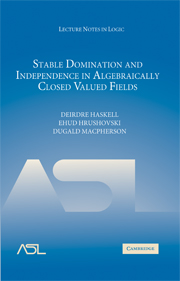# Lecture Notes in Logic, 30

## Stable Domination and Independence in Algebraically Closed Valued Fields

Deirdre Haskell, Ehud Hrushovski, Dugald MacphersonYear: 2007

ISBN-13:9780521889810
194 pages. Hardcover.
Buy now (when ordering, include the discount code ASL2016 to receive the 25% ASL member discount)

This book addresses a gap in the model-theoretic understanding of valued fields that has, until now, limited the interactions of model theory with geometry. It contains significant developments in both pure and applied model theory. Part one of the book is a study of stably dominated types and it begins with an introduction to the key ideas of stability theory for stably dominated types. Part two continues with an outline of some classical results in the model theory of valued fileds and explores the application of stable domination to algebraically closed valued fileds. The research presented here is made accessible to the general model theorist by the inclusion of the introductory sections of each part.

• Introduction

Part I. Stable Domination:

1. Some background on stability theory
2. Definition and basic properties of Stc
3. Invariant types and change of base
4. A combinatorial lemma
5. Strong codes for germs

Part II. Independence in ACVF:

1. Some background on algebraically closed valued fields
2. Sequential independence
3. Growth of the stable part
4. Types orthogonal to \Gamma
5. Opacity and prime resolutions
6. Maximally complete fields and domination
7. Invariant types
8. A maximum modulus principle
9. Canonical bases and independence give by modules
10. Other Henselian fields